Theory and Modern Applications

# Controllability for Sobolev type fractional integro-differential systems in a Banach space

## Abstract

In this paper, by using compact semigroups and the Schauder fixed-point theorem, we study the sufficient conditions for controllability of Sobolev type fractional integro-differential systems in a Banach space. An example is provided to illustrate the obtained results.

MSC:26A33, 34G20, 93B05.

## 1 Introduction

A Sobolev-type equation appears in a variety of physical problems such as flow of fluids through fissured rocks, thermodynamics and propagation of long waves of small amplitude (see ). Recently, there has been an increasing interest in studying the problem of controllability of Sobolev type integro-differential systems. Balachandran and Dauer  studied the controllability of Sobolev type integro-differential systems in Banach spaces. Balachandran and Sakthivel  studied the controllability of Sobolev type semilinear integro-differential systems in Banach spaces. Balachandran, Anandhi and Dauer  studied the boundary controllability of Sobolev type abstract nonlinear integro-differential systems.

In this paper, we study the controllability of Sobolev type fractional integro-differential systems in Banach spaces in the following form: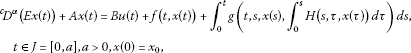(1.1)

where E and A are linear operators with domain contained in a Banach space X and ranges contained in a Banach space Y. The control function $u\left(\cdot \right)$ is in ${L}^{2}\left(J,U\right)$, a Banach space of admissible control functions, with U as a Banach space. B is a bounded linear operator from U into Y. The nonlinear operators $f\in C\left(J×X,Y\right)$, $H\in C\left(J×J×X,X\right)$ and $g\in C\left(J×J×X×X,Y\right)$ are all uniformly bounded continuous operators. The fractional derivative ${}^{c}D^{\alpha }$, $0<\alpha <1$ is understood in the Caputo sense.

## 2 Preliminaries

In this section, we introduce preliminary facts which are used throughout this paper.

Definition 2.1 (see )

The fractional integral of order $\alpha >0$ with the lower limit zero for a function f can be defined as

${I}^{\alpha }f\left(t\right)=\frac{1}{\mathrm{\Gamma }\left(\alpha \right)}{\int }_{0}^{t}\frac{f\left(s\right)}{{\left(t-s\right)}^{1-\alpha }}\phantom{\rule{0.2em}{0ex}}ds,\phantom{\rule{1em}{0ex}}t>0$

provided the right-hand side is pointwise defined on $\left[0,\mathrm{\infty }\right)$, where Γ is the gamma function.

Definition 2.2 (see )

The Caputo derivative of order α with the lower limit zero for a function f can be written as

${}^{c}D^{\alpha }f\left(t\right)=\frac{1}{\mathrm{\Gamma }\left(n-\alpha \right)}{\int }_{0}^{t}\frac{{f}^{\left(n\right)}\left(s\right)}{{\left(t-s\right)}^{\alpha +1-n}}\phantom{\rule{0.2em}{0ex}}ds={I}^{n-\alpha }{f}^{\left(n\right)}\left(t\right),\phantom{\rule{1em}{0ex}}t>0,0\le n-1<\alpha

If f is an abstract function with values in X, then the integrals appearing in the above definitions are taken in Bochner’s sense.

The operators $A:D\left(A\right)\subset X\to Y$ and $E:D\left(E\right)\subset X\to Y$ satisfy the following hypotheses:

$\left({H}_{1}\right)$ A and E are closed linear operators,

$\left({H}_{2}\right)$ $D\left(E\right)\subset D\left(A\right)$ and E is bijective,

$\left({H}_{3}\right)$ ${E}^{-1}:Y\to D\left(E\right)$ is continuous.

The hypotheses ${H}_{1}$, ${H}_{2}$ and the closed graph theorem imply the boundedness of the linear operator $A{E}^{-1}:Y\to Y$.

$\left({H}_{4}\right)$ For each $t\in \left[0,a\right]$ and for some $\lambda \in \rho \left(-A{E}^{-1}\right)$, the resolvent set of $-A{E}^{-1}$, the resolvent $R\left(\lambda ,-A{E}^{-1}\right)$ is a compact operator.

Lemma 2.1 

Let $S\left(t\right)$ be a uniformly continuous semigroup. If the resolvent set $R\left(\lambda ;A\right)$ of A is compact for every $\lambda \in \rho \left(A\right)$, then $S\left(t\right)$ is a compact semigroup.

From the above fact, $-A{E}^{-1}$ generates a compact semigroup $\left\{T\left(t\right),t\ge 0\right\}$ in Y, which means that there exists $M>1$ such that

$\underset{t\in J}{max}\parallel T\left(t\right)\parallel \le M.$
(2.1)

Definition 2.3 The system (1.1) is said to be controllable on the interval J if for every ${x}_{0},{x}_{1}\in X$, there exists a control $u\in {L}^{2}\left(J,U\right)$ such that the solution $x\left(\cdot \right)$ of (1.1) satisfies $x\left(a\right)={x}_{1}$.

$\left({H}_{5}\right)$ The linear operator W from U into X defined by

$Wu={\int }_{0}^{a}{E}^{-1}{\left(a-s\right)}^{\alpha -1}{T}_{\alpha }\left(a-s\right)Bu\left(s\right)\phantom{\rule{0.2em}{0ex}}ds$

has an inverse bounded operator ${W}^{-1}$ which takes values in ${L}^{2}\left(J,U\right)/kerW$, where the kernel space of W is defined by $kerW=\left\{x\in {L}^{2}\left(J,U\right):Wx=0\right\}$, B is a bounded linear operator and ${T}_{\alpha }\left(t\right)$ is defined later.

$\left({H}_{6}\right)$ The function f satisfies the following two conditions:

1. (i)

For each $t\in J$, the function $f\left(t,\cdot \right):X\to Y$ is continuous, and for each $x\in X$, the function $f\left(\cdot ,x\right):J\to Y$ is strongly measurable.

2. (ii)

For each positive number $k\in N$, there is a positive function ${g}_{k}\left(\cdot \right):\left[0,a\right]\to {R}^{+}$ such that

$\underset{|x|\le k}{sup}|f\left(t,x\right)|\le {g}_{k}\left(t\right),$

the function $s\to {\left(t-s\right)}^{1-\alpha }{g}_{k}\left(s\right)\in {L}^{1}\left(\left[0,t\right],{R}^{+}\right)$, and there exists a $\beta >0$ such that

$\underset{k\to \mathrm{\infty }}{lim}inf\frac{{\int }_{0}^{t}{\left(t-s\right)}^{1-\alpha }{g}_{k}\left(s\right)\phantom{\rule{0.2em}{0ex}}ds}{k}=\beta <\mathrm{\infty },\phantom{\rule{1em}{0ex}}t\in \left[0,a\right].$

$\left({H}_{7}\right)$ For each $\left(t,s\right)\in J×J$, the function $H\left(t,s,\cdot \right):X\to X$ is continuous, and for each $x\in X$, the function $H\left(\cdot ,\cdot ,x\right):J×J\to X$ is strongly measurable.

$\left({H}_{8}\right)$ The function g satisfies the following two conditions:

1. (i)

For each $\left(t,s,x\right)\in J×J×X$, the function $g\left(t,s,\cdot ,\cdot \right):X×X\to Y$ is continuous, and for each $x\in X$, $H\in X$, the function $g\left(\cdot ,x,y\right):J×J\to Y$ is strongly measurable.

2. (ii)

For each positive number $k\in N$, there is a positive function ${h}_{k}\left(\cdot \right):\left[0,a\right]\to {R}^{+}$ such that

$\underset{|x|\le k}{sup}|{\int }_{0}^{t}g\left(t,s,x,{\int }_{0}^{s}H\left(s,\tau ,x\right)\phantom{\rule{0.2em}{0ex}}d\tau \right)\phantom{\rule{0.2em}{0ex}}ds|\le {h}_{k}\left(t\right),$

the function $s\to {\left(t-s\right)}^{1-\alpha }{h}_{k}\left(s\right)\in {L}^{1}\left(\left[0,t\right],{R}^{+}\right)$, and there exists a $\gamma >0$ such that

$\underset{k\to \mathrm{\infty }}{lim}inf\frac{{\int }_{0}^{t}{\left(t-s\right)}^{1-\alpha }{h}_{k}\left(s\right)\phantom{\rule{0.2em}{0ex}}ds}{k}=\gamma <\mathrm{\infty },\phantom{\rule{1em}{0ex}}t\in \left[0,a\right].$

According to [11, 12], a solution of equation (1.1) can be represented by

$\begin{array}{rcl}x\left(t\right)& =& {E}^{-1}{S}_{\alpha }\left(t\right)E{x}_{0}+{\int }_{0}^{t}{\left(t-s\right)}^{\alpha -1}{T}_{\alpha }\left(t-s\right){E}^{-1}f\left(s,x\left(s\right)\right)\phantom{\rule{0.2em}{0ex}}ds\\ +{\int }_{0}^{t}{\left(t-s\right)}^{\alpha -1}{E}^{-1}{T}_{\alpha }\left(t-s\right)Bu\left(s\right)\phantom{\rule{0.2em}{0ex}}ds\\ +{\int }_{0}^{t}{\left(t-s\right)}^{\alpha -1}{E}^{-1}{T}_{\alpha }\left(t-s\right)\left\{{\int }_{0}^{s}g\left(s,\tau ,x\left(\tau \right),R\left(\tau \right)\right)\phantom{\rule{0.2em}{0ex}}d\tau \right\}\phantom{\rule{0.2em}{0ex}}ds,\phantom{\rule{1em}{0ex}}t\in J,\end{array}$
(2.2)

where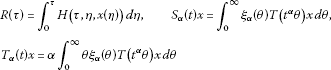with ${\xi }_{\alpha }$ being a probability density function defined on $\left(0,\mathrm{\infty }\right)$, that is, ${\xi }_{\alpha }\left(\theta \right)\ge 0$, $\theta \in \left(0,\mathrm{\infty }\right)$ and ${\int }_{0}^{\mathrm{\infty }}{\xi }_{\alpha }\left(\theta \right)\phantom{\rule{0.2em}{0ex}}d\theta =1$.

Remark ${\int }_{0}^{\mathrm{\infty }}\theta {\xi }_{\alpha }\left(\theta \right)\phantom{\rule{0.2em}{0ex}}d\theta =\frac{1}{\mathrm{\Gamma }\left(1+\alpha \right)}$.

Definition 2.4 By a mild solution of the problem (1.1), we mean that the function $x\in C\left(J,X\right)$ satisfies the integral equation (2.2).

Lemma 2.2 (see )

The operators ${S}_{\alpha }\left(t\right)$ and ${T}_{\alpha }\left(t\right)$ have the following properties:

1. (I)

For any fixed $x\in X$, $\parallel {S}_{\alpha }\left(t\right)x\parallel \le M\parallel x\parallel$, $\parallel {T}_{\alpha }\left(t\right)x\parallel \le \frac{\alpha M}{\mathrm{\Gamma }\left(\alpha +1\right)}\parallel x\parallel$;

2. (II)

$\left\{{S}_{\alpha }\left(t\right),t\ge 0\right\}$ and $\left\{{T}_{\alpha }\left(t\right),t\ge 0\right\}$ are strongly continuous;

3. (III)

For every $t>0$, ${S}_{\alpha }\left(t\right)$ and ${T}_{\alpha }\left(t\right)$ are also compact operators if $T\left(t\right)$, $t>0$ is compact.

## 3 Controllability result

In this section, we present and prove our main result.

Theorem 3.1 If the assumptions $\left({H}_{1}\right)$-$\left({H}_{8}\right)$ are satisfied, then the system (1.1) is controllable on J provided that $\frac{\alpha M\parallel {E}^{-1}\parallel }{\mathrm{\Gamma }\left(\alpha +1\right)}\left(\beta +\gamma \right)\left[1+\frac{{a}^{\alpha }M\parallel {E}^{-1}\parallel }{\mathrm{\Gamma }\left(\alpha +1\right)}\parallel B\parallel \parallel {W}^{-1}\parallel \right]<1$.

Proof Using the assumption $\left({H}_{5}\right)$, for an arbitrary function $x\left(\cdot \right)$, define the control

$\begin{array}{rcl}u\left(t\right)& =& {W}^{-1}\left[{x}_{1}-{E}^{-1}{S}_{\alpha }\left(t\right)E{x}_{0}-{\int }_{0}^{a}{\left(a-s\right)}^{\alpha -1}{E}^{-1}{T}_{\alpha }\left(a-s\right)f\left(s,x\left(s\right)\right)\phantom{\rule{0.2em}{0ex}}ds\\ -{\int }_{0}^{a}{\left(a-s\right)}^{\alpha -1}{E}^{-1}{T}_{\alpha }\left(a-s\right)\left\{{\int }_{0}^{s}g\left(s,\tau ,x\left(\tau \right),R\left(\tau \right)\right)\phantom{\rule{0.2em}{0ex}}d\tau \right\}\phantom{\rule{0.2em}{0ex}}ds\right]\left(t\right).\end{array}$

It shall now be shown that when using this control, the operator Q defined by

$\begin{array}{rcl}\left(Qx\right)\left(t\right)& =& {E}^{-1}{S}_{\alpha }\left(t\right)E{x}_{0}+{\int }_{0}^{t}{\left(t-s\right)}^{\alpha -1}{E}^{-1}{T}_{\alpha }\left(t-s\right)f\left(s,x\left(s\right)\right)\phantom{\rule{0.2em}{0ex}}ds\\ +{\int }_{0}^{t}{\left(t-s\right)}^{\alpha -1}{E}^{-1}{T}_{\alpha }\left(t-s\right)Bu\left(s\right)\phantom{\rule{0.2em}{0ex}}ds\\ +{\int }_{0}^{t}{\left(t-s\right)}^{\alpha -1}{E}^{-1}{T}_{\alpha }\left(t-s\right)\left\{{\int }_{0}^{s}g\left(s,\tau ,x\left(\tau \right),R\left(\tau \right)\right)\phantom{\rule{0.2em}{0ex}}d\tau \right\}\phantom{\rule{0.2em}{0ex}}ds\end{array}$

from $C\left(J,X\right)$ into itself for each $x\in C=C\left(J,X\right)$ has a fixed point. This fixed point is then a solution of equation (2.2).

$\begin{array}{rcl}\left(Qx\right)\left(a\right)& =& {E}^{-1}{S}_{\alpha }\left(a\right)E{x}_{0}+{\int }_{0}^{a}{\left(a-s\right)}^{\alpha -1}{E}^{-1}{T}_{\alpha }\left(a-s\right)f\left(s,x\left(s\right)\right)\phantom{\rule{0.2em}{0ex}}ds\\ +{\int }_{0}^{a}{\left(a-s\right)}^{\alpha -1}{E}^{-1}{T}_{\alpha }\left(a-s\right)B{W}^{-1}\\ ×\left[{x}_{1}-{E}^{-1}{S}_{\alpha }\left(a\right)E{x}_{0}-{\int }_{0}^{a}{\left(a-\tau \right)}^{\alpha -1}{E}^{-1}{T}_{\alpha }\left(a-\tau \right)f\left(\tau ,x\left(\tau \right)\right)\phantom{\rule{0.2em}{0ex}}d\tau \\ -{\int }_{0}^{a}{\left(a-\tau \right)}^{\alpha -1}{E}^{-1}{T}_{\alpha }\left(a-\tau \right)\left\{{\int }_{0}^{\tau }g\left(\tau ,\eta ,x\left(\eta \right),R\left(\eta \right)\right)\phantom{\rule{0.2em}{0ex}}d\eta \right\}\phantom{\rule{0.2em}{0ex}}d\tau \right]\left(s\right)\phantom{\rule{0.2em}{0ex}}ds\\ +\alpha {\int }_{0}^{a}{\left(a-s\right)}^{\alpha -1}\phantom{\rule{0.25em}{0ex}}{E}^{-1}{T}_{\alpha }\left(a-s\right)\left\{{\int }_{0}^{s}g\left(s,\tau ,x\left(\tau \right),R\left(\tau \right)\right)\phantom{\rule{0.2em}{0ex}}d\tau \right\}\phantom{\rule{0.2em}{0ex}}ds={x}_{1}.\end{array}$

It can be easily verified that Q maps C into itself continuously.

For each positive number $k>0$, let ${B}_{k}=\left\{x\in C:x\left(0\right)={x}_{0},\parallel x\left(t\right)\parallel \le k,t\in J\right\}$. Obviously, ${B}_{k}$ is clearly a bounded, closed, convex subset in C. We claim that there exists a positive number k such that $Q{B}_{k}\subset {B}_{k}$. If this is not true, then for each positive number k, there exists a function ${x}_{k}\in {B}_{k}$ with $Q{x}_{k}\notin {B}_{k}$, that is, $\parallel Q{x}_{k}\parallel \ge k$, then $1\le \frac{1}{k}\parallel Q{x}_{k}\parallel$, and so

$1\phantom{\rule{0.25em}{0ex}}\le \underset{k\to \mathrm{\infty }}{lim}{k}^{-1}\parallel Q{x}_{k}\parallel .$

However,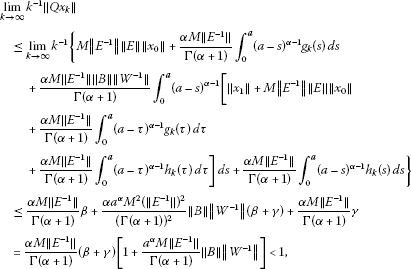a contradiction. Hence, $Q{B}_{k}\subset {B}_{k}$ for some positive number k. In fact, the operator Q maps ${B}_{k}$ into a compact subset of ${B}_{k}$. To prove this, we first show that the set ${V}_{k}\left(t\right)=\left\{\left(Qx\right)\left(t\right):x\in {B}_{k}\right\}$ is a precompact in X; for every $t\in J$: This is trivial for $t=0$, since ${V}_{k}\left(0\right)=\left\{{x}_{0}\right\}$. Let t, $0; be fixed. For $0<ϵ and arbitrary $\delta >0$; take

$\begin{array}{rcl}\left({Q}^{ϵ,\delta }x\right)\left(t\right)& =& {\int }_{\delta }^{\mathrm{\infty }}{\xi }_{\alpha }\left(\theta \right){E}^{-1}T\left({t}^{\alpha }\theta \right)E{x}_{0}\phantom{\rule{0.2em}{0ex}}d\theta \\ +\alpha {\int }_{0}^{t-ϵ}{\int }_{\delta }^{\mathrm{\infty }}\theta {\left(t-s\right)}^{\alpha -1}{\xi }_{\alpha }\left(\theta \right){E}^{-1}T\left({\left(t-s\right)}^{\alpha }\theta \right)f\left(s,x\left(s\right)\right)\phantom{\rule{0.2em}{0ex}}d\theta \phantom{\rule{0.2em}{0ex}}ds\\ +\alpha {\int }_{0}^{t-ϵ}{\int }_{\delta }^{\mathrm{\infty }}\theta {\left(t-s\right)}^{\alpha -1}{\xi }_{\alpha }\left(\theta \right){E}^{-1}T\left({\left(t-s\right)}^{\alpha }\theta \right)\\ ×B{W}^{-1}\left[{x}_{1}-{\int }_{0}^{\mathrm{\infty }}{\xi }_{\alpha }\left(\theta \right){E}^{-1}T\left({a}^{\alpha }\theta \right)E{x}_{0}\phantom{\rule{0.2em}{0ex}}d\theta \\ -\alpha {\int }_{0}^{a}{\int }_{0}^{\mathrm{\infty }}\theta {\left(a-\tau \right)}^{\alpha -1}{\xi }_{\alpha }\left(\theta \right){E}^{-1}T\left({\left(a-\tau \right)}^{\alpha }\theta \right)f\left(\tau ,x\left(\tau \right)\right)\phantom{\rule{0.2em}{0ex}}d\theta \phantom{\rule{0.2em}{0ex}}d\tau \\ -\alpha {\int }_{0}^{a}{\int }_{0}^{\mathrm{\infty }}\theta {\left(a-\tau \right)}^{\alpha -1}{\xi }_{\alpha }\left(\theta \right){E}^{-1}T\left({\left(a-\tau \right)}^{\alpha }\theta \right)\\ ×\left\{{\int }_{0}^{\tau }g\left(\tau ,\eta ,x\left(\eta \right),R\left(\eta \right)\right)\phantom{\rule{0.2em}{0ex}}d\eta \right\}\phantom{\rule{0.2em}{0ex}}d\theta \phantom{\rule{0.2em}{0ex}}d\tau \right]\left(s\right)\phantom{\rule{0.2em}{0ex}}d\theta \phantom{\rule{0.2em}{0ex}}ds\\ +\alpha {\int }_{0}^{t-ϵ}{\int }_{\delta }^{\mathrm{\infty }}\theta {\left(t-s\right)}^{\alpha -1}{\xi }_{\alpha }\left(\theta \right){E}^{-1}T\left({\left(t-s\right)}^{\alpha }\theta \right)\\ ×\left\{{\int }_{0}^{s}g\left(s,\tau ,x\left(\tau \right),R\left(\tau \right)\right)\phantom{\rule{0.2em}{0ex}}d\tau \right\}\phantom{\rule{0.2em}{0ex}}d\theta \phantom{\rule{0.2em}{0ex}}ds\\ =& T\left({ϵ}^{\alpha }\delta \right){\int }_{\delta }^{\mathrm{\infty }}{\xi }_{\alpha }\left(\theta \right){E}^{-1}T\left({t}^{\alpha }\theta -{ϵ}^{\alpha }\delta \right)E{x}_{0}\phantom{\rule{0.2em}{0ex}}d\theta \\ +T\left({ϵ}^{\alpha }\delta \right)\alpha {\int }_{0}^{t-ϵ}{\int }_{\delta }^{\mathrm{\infty }}\theta {\left(t-s\right)}^{\alpha -1}{\xi }_{\alpha }\left(\theta \right){E}^{-1}T\left({\left(t-s\right)}^{\alpha }\theta -{ϵ}^{\alpha }\delta \right)f\left(s,x\left(s\right)\right)\phantom{\rule{0.2em}{0ex}}d\theta \phantom{\rule{0.2em}{0ex}}ds\\ +T\left({ϵ}^{\alpha }\delta \right)\alpha {\int }_{0}^{t-ϵ}{\int }_{\delta }^{\mathrm{\infty }}\theta {\left(t-s\right)}^{\alpha -1}{\xi }_{\alpha }\left(\theta \right){E}^{-1}T\left({\left(t-s\right)}^{\alpha }\theta -{ϵ}^{\alpha }\delta \right)\\ ×B{W}^{-1}\left[{x}_{1}-{\int }_{0}^{\mathrm{\infty }}{\xi }_{\alpha }\left(\theta \right){E}^{-1}T\left({a}^{\alpha }\theta \right)E{x}_{0}\phantom{\rule{0.2em}{0ex}}d\theta \\ -\alpha {\int }_{0}^{a}{\int }_{0}^{\mathrm{\infty }}\theta {\left(a-\tau \right)}^{\alpha -1}{\xi }_{\alpha }\left(\theta \right){E}^{-1}T\left({\left(a-\tau \right)}^{\alpha }\theta \right)f\left(\tau ,x\left(\tau \right)\right)\phantom{\rule{0.2em}{0ex}}d\theta \phantom{\rule{0.2em}{0ex}}d\tau \\ -{\int }_{0}^{a}{\int }_{0}^{\mathrm{\infty }}\theta {\left(a-\tau \right)}^{\alpha -1}{\xi }_{\alpha }\left(\theta \right){E}^{-1}T\left({\left(a-\tau \right)}^{\alpha }\theta \right)\\ ×\left\{{\int }_{0}^{\tau }g\left(\tau ,\eta ,x\left(\eta \right),R\left(\eta \right)\right)\phantom{\rule{0.2em}{0ex}}d\eta \right\}\phantom{\rule{0.2em}{0ex}}d\theta \phantom{\rule{0.2em}{0ex}}d\tau \right]\left(s\right)\phantom{\rule{0.2em}{0ex}}d\theta \phantom{\rule{0.2em}{0ex}}ds\\ +T\left({ϵ}^{\alpha }\delta \right)\alpha {\int }_{0}^{t-ϵ}{\int }_{\delta }^{\mathrm{\infty }}\theta {\left(t-s\right)}^{\alpha -1}{\xi }_{\alpha }\left(\theta \right){E}^{-1}T\left({\left(t-s\right)}^{\alpha }\theta -{ϵ}^{\alpha }\delta \right)\\ ×\left\{{\int }_{0}^{s}g\left(s,\tau ,x\left(\tau \right),R\left(\tau \right)\right)\phantom{\rule{0.2em}{0ex}}d\tau \right\}\phantom{\rule{0.2em}{0ex}}d\theta \phantom{\rule{0.2em}{0ex}}ds.\end{array}$

Since $u\left(s\right)$ is bounded and $T\left({ϵ}^{\alpha }\delta \right)$, ${ϵ}^{\alpha }\delta >0$ is a compact operator, then the set ${V}_{k}^{ϵ,\delta }\left(t\right)=\left\{\left({Q}^{ϵ,\delta }x\right)\left(t\right):x\in {B}_{k}\right\}$ is a precompact set in X for every ϵ, $0<ϵ, and for all $\delta >0$. Also, for $x\in {B}_{k}$, using the defined control $u\left(t\right)$ yields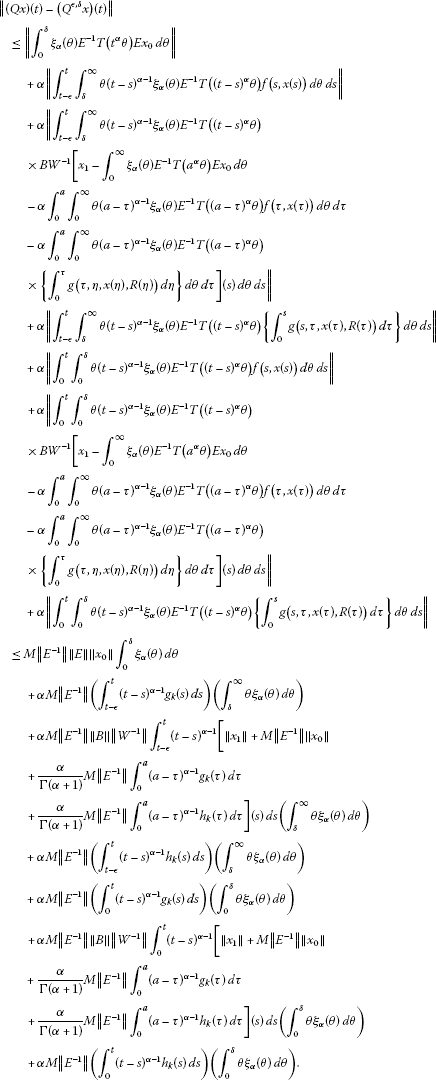Therefore, as $ϵ\to {0}^{+}$ and $\delta \to {0}^{+}$, there are precompact sets arbitrary close to the set ${V}_{k}\left(t\right)$ and so ${V}_{k}\left(t\right)$ is precompact in X.

Next, we show that $Q{B}_{k}=\left\{Qx:x\in {B}_{k}\right\}$ is an equicontinuous family of functions.

Let $x\in {B}_{k}$ and $t,\tau \in J$ such that $0, then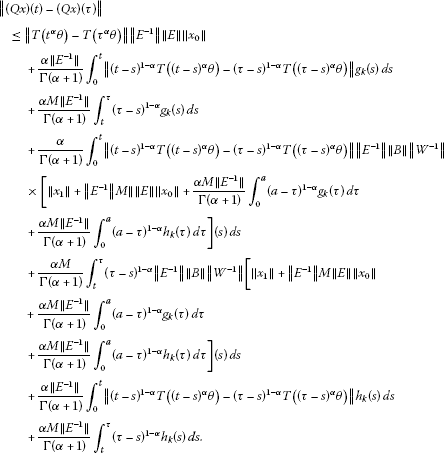Now, $T\left(t\right)$ is continuous in the uniform operator topology for $t>0$ since $T\left(t\right)$ is compact, and the right-hand side of the above inequality tends to zero as $t\to \tau$. Thus, $Q{B}_{k}$ is both equicontinuous and bounded. By the Arzela-Ascoli theorem, $Q{B}_{k}$ is precompact in $C\left(J,X\right)$. Hence, Q is a completely continuous operator on $C\left(J,X\right)$.

From the Schauder fixed-point theorem, Q has a fixed point in ${B}_{k}$. Any fixed point of Q is a mild solution of (1.1) on J satisfying $\left(Qx\right)\left(t\right)=x\left(t\right)\in X$. Thus, the system (1.1) is controllable on J. □

## 4 Example

In this section, we present an example to our abstract results.

We consider the fractional integro-partial differential equation in the form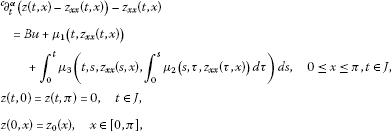(4.1)

where ${}^{c}\partial _{t}^{\alpha }$ is the Caputo fractional partial derivative of order $0<\alpha <1$.

Take $X=Y={L}^{2}\left[0,\pi \right]$ and define the operators $A:D\left(A\right)\subset X\to Y$ and $E:D\left(E\right)\subset X\to Y$ by $Az=-{z}_{xx}$ and $Ez=z-{z}_{xx}$, where each domain $D\left(A\right)$ and $D\left(E\right)$ is given by .

Then A and E can be written respectively as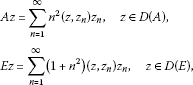where ${z}_{n}\left(x\right)=\sqrt{2/\pi }sinnx$, $n=1,2,\dots$ , is the orthonormal set of eigenvectors of A and $\left(z,{z}_{n}\right)$ is the ${L}^{2}$ inner product. Moreover, for $z\in X$, we get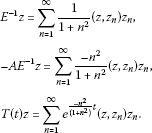We assume that

$\left({A}_{1}\right)$: The operator $B:U\to Y$, with $U\subset J$, is a bounded linear operator.

$\left({A}_{2}\right)$: The linear operator $W:U\to X$ defined by

$Wu={\int }_{0}^{a}{E}^{-1}{\left(a-s\right)}^{\alpha -1}{T}_{\alpha }\left(a-s\right)Bu\left(s\right)\phantom{\rule{0.2em}{0ex}}ds$

has an inverse bounded operator ${W}^{-1}$ which takes values in ${L}^{2}\left(J,U\right)/kerW$, where the kernel space of W is defined by $kerW=\left\{x\in {L}^{2}\left(J,U\right):Wx=0\right\}$, B is a bounded linear operator.

$\left({A}_{3}\right)$: The nonlinear operator ${\mu }_{1}:J×X\to Y$ satisfies the following three conditions:

1. (i)

For each $t\in J$, ${\mu }_{1}\left(t,z\right)$ is continuous.

2. (ii)

For each $z\in X$, ${\mu }_{1}\left(t,z\right)$ is measurable.

3. (iii)

There is a constant ν ($0<\nu <1$) and a function $h\left(\cdot \right):\left[0,a\right]\to {R}^{+}$ such that for all $\left(t,z\right)\in J×X$,

$\parallel {\mu }_{1}\left(t,z\right)\parallel \le h\left(t\right){|z|}^{\nu }.$

$\left({A}_{4}\right)$: The nonlinear operator ${\mu }_{2}:J×J×X\to X$ satisfies the following two conditions:

1. (i)

For each $\left(t,s\right)\in J×J$, ${\mu }_{2}\left(t,s,z\right)$ is continuous.

2. (ii)

For each $z\in X$, ${\mu }_{2}\left(t,s,z\right)$ is measurable.

$\left({A}_{5}\right)$: The nonlinear operator ${\mu }_{3}:J×J×X×X\to Y$ satisfies the following three conditions:

1. (i)

For each $\left(t,s,z\right)\in J×J×X$, ${\mu }_{3}\left(t,s,z\right)$ is continuous.

2. (ii)

For each $z\in X$, ${\mu }_{3}\left(t,s,z\right)$ is measurable.

3. (iii)

There is a constant ν ($0<\nu <1$) and a function $g\left(\cdot \right):\left[0,a\right]\to {R}^{+}$ such that for all $\left(t,s,z,y\right)\in J×J×X×X$,

$\parallel {\int }_{0}^{t}{\mu }_{3}\left(t,s,z,{\int }_{0}^{s}{\mu }_{2}\left(s,\tau ,z\right)\phantom{\rule{0.2em}{0ex}}d\tau \right)\phantom{\rule{0.2em}{0ex}}ds\parallel \le g\left(t\right){|z|}^{\nu }.$

Define an operator $f:J×X\to Y$ by

$f\left(t,z\right)\left(x\right)={\mu }_{1}\left(t,{z}_{xx}\left(x\right)\right)$

and let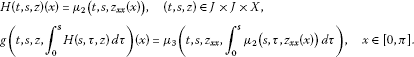Then the problem (4.1) can be formulated abstractly as: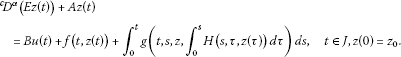It is easy to see that $-A{E}^{-1}$ generates a uniformly continuous semigroup ${\left\{S\left(t\right)\right\}}_{t\ge 0}$ on Y which is compact, and (2.1) is satisfied. Also, the operator f satisfies condition $\left({H}_{6}\right)$ and the operator H and g satisfy $\left({H}_{7}\right)$ and $\left({H}_{8}\right)$. Also all the conditions of Theorem 3.1 are satisfied. Hence, the equation (4.1) is controllable on J.

## References

1. Barenblatt G, Zheltov I, Kochina I: Basic concepts in the theory of seepage of homogeneous liquids in fissured rocks. J. Appl. Math. Mech. 1960, 24: 1286–1303. 10.1016/0021-8928(60)90107-6

2. Chen PJ, Curtin ME: On a theory of heat conduction involving two temperatures. Z. Angew. Math. Phys. 1968, 19: 614–627. 10.1007/BF01594969

3. Huilgol R: A second order fluid of the differential type. Int. J. Non-Linear Mech. 1968, 3: 471–482. 10.1016/0020-7462(68)90032-2

4. Balachandran K, Dauer JP: Controllability of Sobolev type integrodifferential systems in Banach spaces. J. Math. Anal. Appl. 1998, 217: 335–348. 10.1006/jmaa.1997.5725

5. Balachandran K, Sakthivel R: Controllability of Sobolev type semilinear integro-differential systems in Banach spaces. Appl. Math. Lett. 1999, 12: 63–71.

6. Balachandran K, Anandhi ER, Dauer JP: Boundary controllability of Sobolev type abstract nonlinear integro-differential systems. J. Math. Anal. Appl. 2003, 277: 446–464. 10.1016/S0022-247X(02)00522-X

7. Podlubny I: Fractional Differential Equations. Academic Press, San Diego; 1999.

8. Miller KS, Ross B: An Introduction to the Fractional Calculus and Fractional Differential Equations. John Wiley, New York; 1993.

9. Samko S, Kilbas A, Marichev OL: Fractional Integrals and Derivatives. Gordon & Breach, New York; 1993.

10. Pazy A: Semigroups of Linear Operators and Applications to Partial Differential Equations. Springer, New York; 1983.

11. Zhou Y, Jiao F: Existence of mild solutions for fractional neutral evolution equations. Comput. Math. Appl. 2010, 59: 1063–1077. 10.1016/j.camwa.2009.06.026

12. El-Borai MM: Some probability densities and fundamental solutions of fractional evolution equations. Chaos Solitons Fractals 2002, 14(3):433–440. 10.1016/S0960-0779(01)00208-9

13. Lightboure JH III, Rankin SM III: A partial functional differential equation of Sobolev type. J. Math. Anal. Appl. 1983, 93: 328–337. 10.1016/0022-247X(83)90178-6

## Acknowledgements

I would like to thank the referees and professor Ravi Agarwal for their valuable comments and suggestions.

## Author information

Authors

### Corresponding author

Correspondence to Hamdy M Ahmed.

### Competing interests

The author declare that he has no competing interests.

## Rights and permissions

Reprints and Permissions

Ahmed, H.M. Controllability for Sobolev type fractional integro-differential systems in a Banach space. Adv Differ Equ 2012, 167 (2012). https://doi.org/10.1186/1687-1847-2012-167

• Accepted:

• Published:

• DOI: https://doi.org/10.1186/1687-1847-2012-167

### Keywords

• fractional calculus
• Sobolev type fractional integro-differential systems
• controllability
• compact semigroup
• mild solution
• Schauder fixed-point theorem